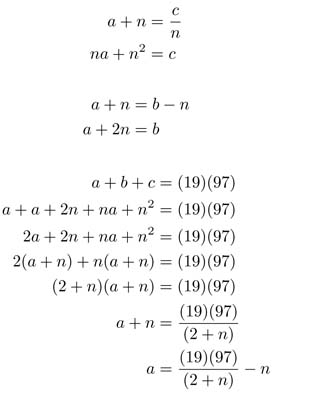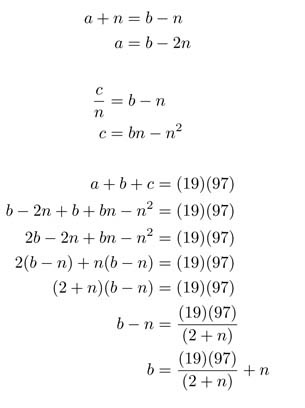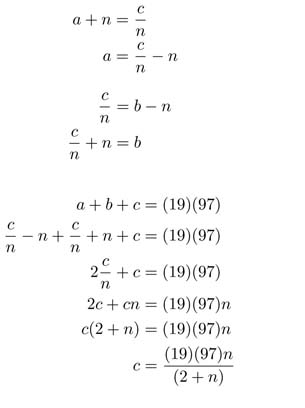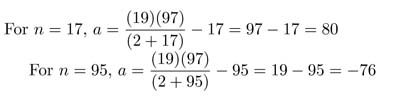SEARCH HOMEMath Central Quandaries & Querieswilliam, a student: let a, b, c and n be positive integers. If a+b+c=(19)(97) and a+n=b-n=c/n, compute the value of a.Hi William,

It is best if you first write a, b & c in terms of n.Looking at c, we know n must either be 17 or 95 or else c is not a positive integer. Now we need to determine whether 17 or 95 will result in a positive integer value for a.The n value of 95 results in a negative a value so we discard this answer so the value of a is 80.

Hope this Helps,

JaniceMath Central is supported by the University of Regina and The Pacific Institute for the Mathematical Sciences.